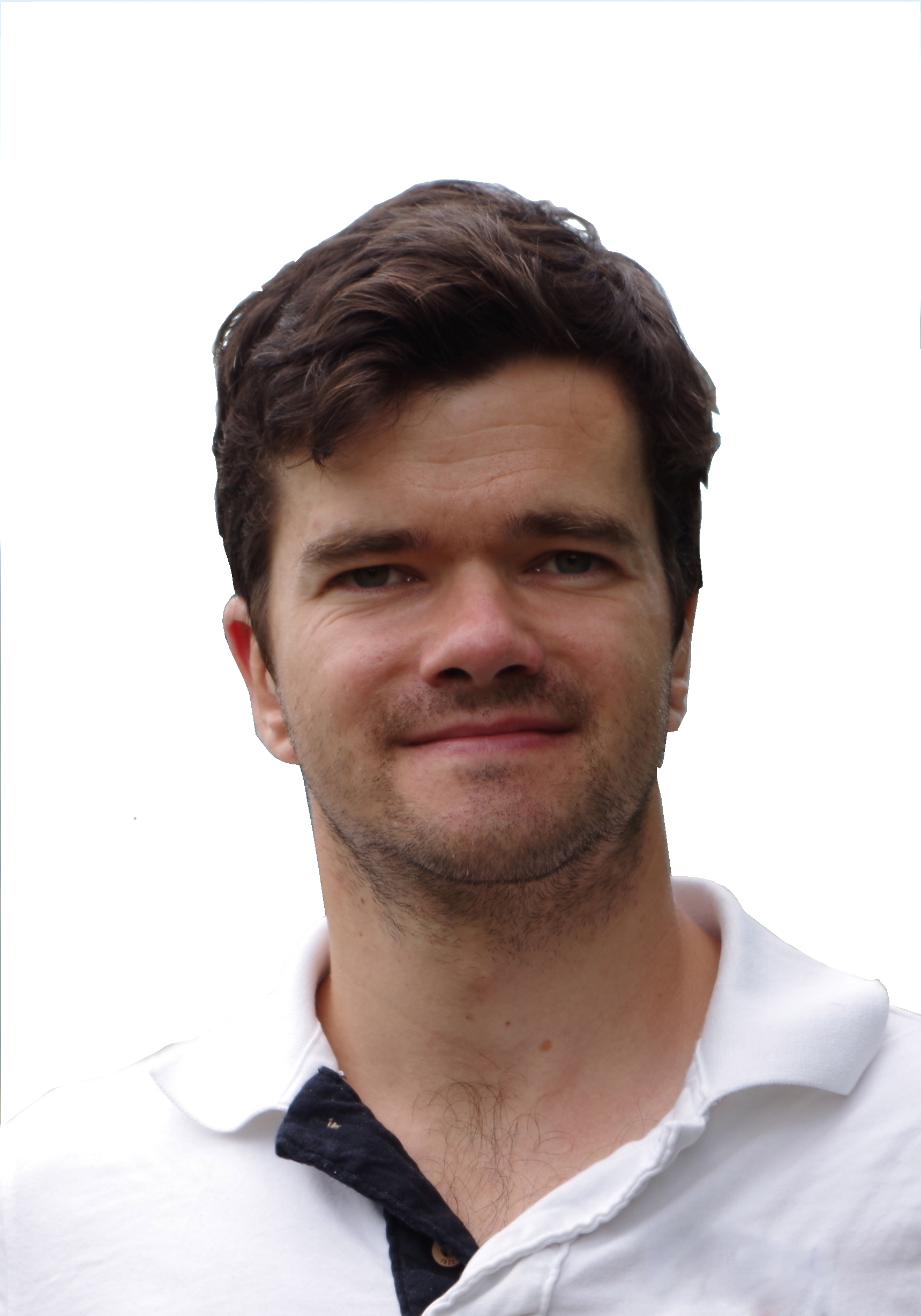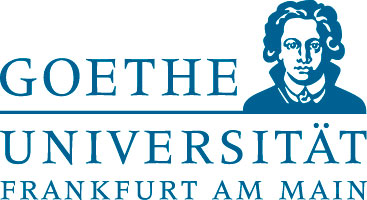# Prof. Dr. Julian Scheuer

## Goethe Universität Frankfurt

Institut für Mathematik
Robert-Mayer-Str. 10, Raum 803
60325 Frankfurt
E-Mail: scheuer@math.uni-frankfurt.de
Phone: +49-69-798-22568
Geometric Analysis and
Partial Differential EquationsEPSRC Small Grant Scheme: Stability for nonlocal curvature functionals, EPSRC:EP/W014807/2

In classical hypersurface theory, a famous class of questions is about stability of objects with respect to pinching of curvature quantities. Exemplarily, the shape of hypersurfaces with almost constant mean curvature has been a property of investigation in the literature. For the nonlocal mean curvature such question were also studied by various authors. This project is supposed to find more natural nonlocal curvature quantities with the potential to control the shape of the hypersurface globally. In doing so, possible new notions of nonlocal curvature and their properties shall be investigated.

EPSRC DTP-Project: Stability in physical systems governed by curvature quantities

We study problems in hypersurface geometry involving curvature constraints. A simple example arises from Alexandrov's soap bubble theorem, which states that every constant mean curvature embedded closed hypersurface must be a round sphere. In this project we study the question of stability: Is an almost constant mean curvature hypersurface necessarily close to a sphere. While this particular problem has been treated extensively by many authors, our project develops new tools which also apply in related problems, such as stability in Alexandrov-Fenchel inequalities, in Serrin's overdetermined problem and in Steklov eigenvalue problems. A first reference is the paper

DFG-Project: Quermassintegral preserving local curvature flows, gepris:400729345

We consider inverse curvature flows with forcing terms of closed graphical and mean-convex hypersurfaces in a class of cylindrical warped ambient manifolds, which contains the simply connected spaceforms. For example, by adding suitable combinations of the radial distance and angle functions to the inverse mean curvature flow equation, one obtains a surface area preserving flow which decreases the total mean curvature. The special feature of this flow is that, contrary to previous quermassintegral preserving flows, it is local and does not contain a nonlocal forcing term. This feature has several technical benefits. The aim of this project is to deduce the long-time existence of these flows and smooth convergence to a coordinate slice for graphical and mean-convex initial hypersurfaces. As applications several generalisations of classical Alexandrov-Fenchel inequalities would follow for non-convex hypersurfaces. A Heintze-Karcher type inequality for closed mean-convex hypersurfaces has proven to be a helpful tool in the deduction of monotone quantities along such flows. This inequality holds with precise equality if and only if the hypersurface is a coordinate slice. A stability version of this result would be very useful in the investigation of the asymptotics of such curvature flows and hence shall also be deduced within this project.

The starting point of this project was the paper

which proves new Minkowski type inequalities in a class of warped product spaces with the help of locally constrained inverse curvature flows. A related result for convex hypersurfaces with free boundary in the unit ball, which covers Alexandrov-Fenchel inequalities for such hypersurfaces, is given in

After those results the main part of the project was carried out at Columbia University. We achieved a variety of results in curvature flows and applications to geometric inequalities, such as Minkowski inequalities in

an isoperimetric inequality for spacelike hypersurfaces in generalised Robertson-Walker spaces

as well as curvature type equations and Orlicz-Minkowski problems in

A stability result for the Heintze-Karcher inequality is deduced, among other things, here:

DFG-Project: Harnack inequalities for curvature flows and applications, gepris:319506420

The object of investigation of this project is the behaviour of strictly convex hypersurfaces of Riemannian manifolds under deformation by their curvature, e.g. under the mean curvature flow and its fully nonlinear variants. We aim to derive Harnack inequalities for the flow speed, a problem which has been treated mostly in the Euclidean space. As applications we aim for new convergence results for flows in the sphere, especially for the classification of ancient solutions and for smooth convergence of suitably rescaled hypersurfaces. These results shall be proven for a class of flow speeds which includes powers of the Gaussian curvature and shall also be treated in manifolds of non-constant sectional curvature. Obtaining a good control of the flow speed is usually a major difficulty in such problems, which shall be overcome with the Harnack inequalities. In order to prove them in a general setting, we aim to find a well-suited substitute for the well-known Gauss parametrization of a strictly convex hypersurface in the Euclidean space. These questions tie in with the current state of research and highlight some unanswered aspects in the theory of curvature flows.

This project emerged from the paper

which provides the first Harnack inequalities for curvatures flows outside the Euclidean space. This initiated a more general study.

The major result within this project is the following preprint, in which we found a suitable replacement for the Gauss map parametrisation of a convex hypersurface in the Euclidean space, which also works in general backgrounds.

As a supplement to the aforementioned paper, in which we faced certain problems with the proper use of curvature functions, the following work emerged.

During the search for Harnack inequalities for inverse curvature flows, we found counterexamples to the preservation of convexity for some particular high power inverse mean curvature flows, making the the deduction of Harnack inequalities for convex solutions to such flows obsolete.

With regards to the application of Harnack inequalities to the classification of ancient solutions to curvature flows, we could provide the following general result about ancient solutions to curvature flows in the sphere.

The first results on Harnack inequalities in Riemannian manifolds lacking local symmetry are provided in the following paper.